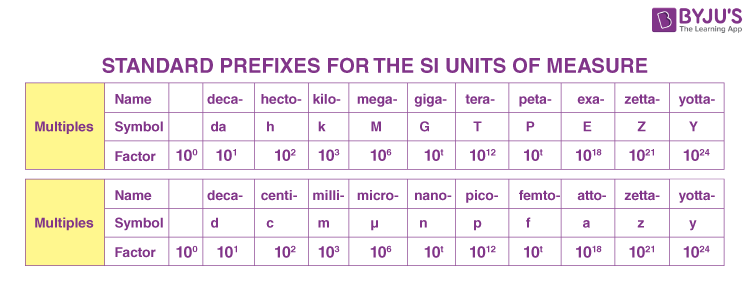# Standard Units of Measurement

A standard unit of measurement is a quantifiable language that describes the magnitude of the quantity. It helps to understand the association of the object with the measurement. Although measurement is an important part of everyday life, kids don’t automatically understand the different ways to measure things. This article will discuss the different units and measurements and why we need them.

## Need For Standard Units and Measurement

There are many systems and units in place for measuring different quantities like length, area, mass, volume and other things. For example, an acre is a common way of representing area measurement in India. One acre is around 4046 square metres, according to the metric system. So now you can guess how difficult it would be if there were no standard units and measurements.

Similarly, the temperature is measured in degree celsius, and the same unit of measurement cannot be used to measure the length of a rod. Each quantity has to be measured in its own way. The magnitude and the measurement vary along with the quantity. Hence the need for the units of measurement for each quantity arises.

## What is the International System of Units?

After learning the importance of units and measurements, let us focus on the International System of Units. Traditionally, people didn’t have any measuring devices to calculate standard measurement units. To tackle this problem, they devised different innovative measuring methods with the available tools. For example, they used a foot as a measurement of length. 1 foot is around 0.3 metres which are 30 cm. Another measurement our ancestors used for length was a league. One league was the distance covered by a person when we walked for an hour. This unit, however, is no longer in use.

To tackle this problem of different systems of measurement (just like the different languages), a system of units called the International System of Units was established and has been adopted by most developed and developing countries. Although this has been established and adopted across major fields like science and technology and government operations, people normally refer to their customary or traditional units. For example, in the United States of America, people even now refer to lengths in terms of inches and feet instead of centimetres and metres.

### International System of Units

The International System of Units or SI units defines standard units for measurement of all physical quantities.
In principle, any physical quantity can be expressed in terms of seven base units.

 Seven Base Units Property Unit Symbol Length metre m Mass kilogram kg Time second s Electric Current Ampere A Temperature Kelvin K Amount of Substance mole mol Luminous Intensity candela cd

### Derived Units

Apart from the base units, there are SI units of derived units. These are called as such because their value is determined based on one or more base units. Some examples are given below.

• Frequency – Hertz (Hz); 1 Hz = 1 s-1
• Power – Watt (W); 1 W = 1 kg·m2s−3

### SI Unit Prefixes

The SI system utilizes a standard system of prefixes to the basic units, allowing them to be more relevant and descriptive of relative magnitude. Prefixes are used to identify the original unit’s multiples or fractions. There are 20 accepted prefixes. The table below lists the standard prefixes for the SI units of measurement.Related Articles:

## Frequently Asked Questions – FAQs

Q1

### Can a quantity have dimensions but no units associated with it?

No. If a quantity has dimensions, then it should have units associated with it.

Q2

### What are the dimensions of a physical quantity?

Dimensions of a physical quantity refer to the nature of the quantity, which defines it in some measurable terms. The physical dimensions are expressed in terms of length, mass and time, represented by L, M and T, respectively.

Q3

### Define a unit

Any given physical quantity is measured compared to a basic unit that is arbitrarily chosen and internationally accepted. The result of a measurement is expressed by a number which is accompanied by a unit.

Q4

### What are supplementary units?

Supplementary units are dimensionless physical quantities that are used along with fundamental units.

Q5

### Give an example of derived units.

Velocity is a physical quantity derived from fundamental quantities of length and time.
The unit of velocity is ms-1.

## Watch the video to find out what the base measurements areHope you have clearly understood the units and measurements. Stay tuned with BYJU’S to learn more about the International System of Units, various derivations, Laws in Physics and much more.

Test your knowledge on Standard Measurement Units